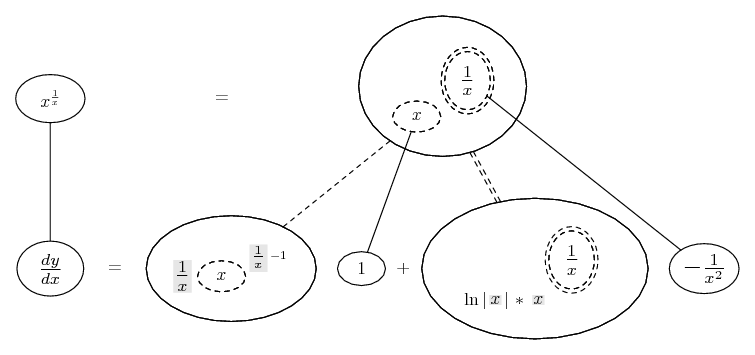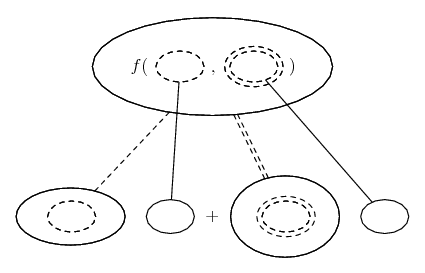# Thread: Derivative of x^(1/x)

1. ## Derivative of x^(1/x)

Hi,

I've been stumped trying to find the derivative $\displaystyle \frac{d}{dx} \left [x^{1/x} \right ]$.

By the chain, rule: $\displaystyle \frac{d}{dx} \left [x^{u} \right ]=ux^{u-1} \frac{du}{dx}$.
Substituting $\displaystyle u=\frac{1}{x}=x^{-1}$ and $\displaystyle \frac{du}{dx}=-x^{-2}$ results in: $\displaystyle \frac{d}{dx} \left [x^{1/x} \right ]=x^{-1} \cdot x^{\frac{1}{x}-1} \cdot -x^{-2}=-x^{\frac{1}{x}-4}$

However, that cannot be the correct derivative. By inspecting their graphs, $\displaystyle x^{1/x}$ appears to have a critical (turning) point, yet there is no solution to $\displaystyle 0=-x^{\frac{1}{x}-4}$.

Is there any reason for this apparent contradiction? Did I make a mistake?
Thanks in advance for any insight on this issue.

2. Use the transform $\displaystyle y = 1/x \cdot \ln x$.

3. logarithmic differentiation ...

$\displaystyle y = x^{\frac{1}{x}}$

$\displaystyle \ln{y} = \frac{1}{x} \ln{x}$

$\displaystyle \frac{y'}{y} = \frac{1}{x^2} - \frac{\ln{x}}{x^2} = \frac{1-\ln{x}}{x^2}$

$\displaystyle y' = y\left(\frac{1-\ln{x}}{x^2}\right) = x^{\frac{1}{x}}\left(\frac{1-\ln{x}}{x^2}\right)$

4. Thanks.

I see how that leads to the right answer, but I don't understand the logic.

Why does the chain rule lie? Also, using logarithmic differentiation places the restriction that x > 0 and y > 0.

5.Originally Posted by Progenitor12Thanks.

I see how that leads to the right answer, but I don't understand the logic.

Why does the chain rule lie? Also, using logarithmic differentiation places the restriction that x > 0 and y > 0.
Because the function composition here is $\displaystyle f(g(x))$ where $\displaystyle f(x)=x^x$ and $\displaystyle g(x)=\frac{1}{x}$. Be careful how you apply the chain rule.

Also, at your level, does it matter if you get an imaginary value if it's sent right back to the real numbers?

6.Originally Posted by Progenitor12Thanks.

I see how that leads to the right answer, but I don't understand the logic.

Why does the chain rule lie? Also, using logarithmic differentiation places the restriction that x > 0 and y > 0.
you cannot use the general power rule for exponential functions.

the derivative of $\displaystyle y = x^u$ is not $\displaystyle ux^{u-1} \cdot u'$

7.Originally Posted by skeeteryou cannot use the general power rule for exponential functions.

the derivative of $\displaystyle y = x^u$ is not $\displaystyle ux^{u-1} \cdot u'$
I think that the distinction that needs to be made is that $\displaystyle \bigg[x^n\bigg]'=nx^{n-1}$ provided $\displaystyle n$ is a constant.

8.Originally Posted by Drexel28Because the function composition here is $\displaystyle f(g(x))$ where $\displaystyle f(x)=x^x$ and $\displaystyle g(x)=\frac{1}{x}$. Be careful how you apply the chain rule.

Also, at your level, does it matter if you get an imaginary value if it's sent right back to the real numbers?
Ah, okay, that makes sense. Now that I think about it, $\displaystyle x^{1/x}$ is discontinuous for y < 0 and x < 0 anyway, so the restrictions don't matter. It's only defined for positive x, and negative values of x that have an odd integer in the numerator when expressed as a fraction in simplest form.

9. Just by the way - - Wolfram|Alpha does it with the chain rule for two inner functions...

$\displaystyle \frac{d}{dx}\ f(u(x), v(x)) = \frac{\partial f}{\partial u} \frac{du}{dx} + \frac{\partial f}{\partial v} \frac{dv}{dx}$

... which doesn't mean we have to, of course, but I fancy this picture makes it palatable...... where... is the double version of...... the ordinary chain rule. As with that, straight continuous lines differentiate downwards (integrate up) with respect to x, and the straight dashed lines similarly but with respect to the (corresponding) dashed balloon expression which is (one of) the inner function(s) of the composite expression.

Shading shows that an expression has been treated as a constant on the way down (during differentiation).

You do, admittedly, need logarithmic differentiation to see the logic of the double-dashed differentiation, i.e. the logic of...

$\displaystyle \frac{d}{du} a^u = \ln a\ a^u$

_____________________________________

Don't integrate - balloontegrate!

Balloon Calculus Forum

Balloon Calculus Drawing with LaTeX and Asymptote!

#### Search Tags

derivative, x1 or x